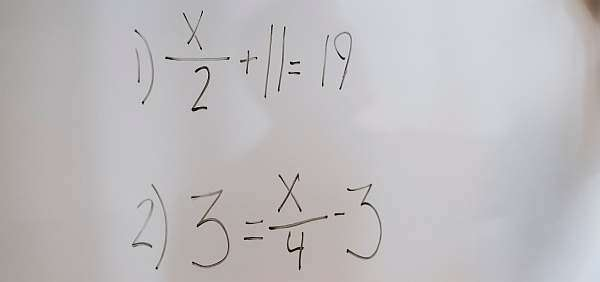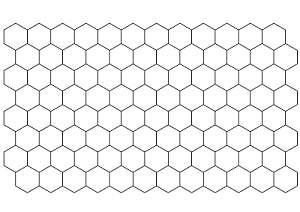# Solve For x Calculator

Instructions: Use this step-by-step calculator to solve for x from a linear equation that you provide. Please type a valid linear equation in the box provided below.Type a linear equation (Ex: 3x - 2y = 3, x - y= 2/3 x, etc.)

Traditionally, you have to solve equations, and the most common setting is to solve equations when there is only one variable.

But it also could be the case that you want to solve an equation when more than one variable is present. In that case, you say that you solve for that variable, as oppose to solving for any other variable present.

The case of a linear equation, with two variables $$x$$ and $$y$$ is one of the simplest cases you can have in which you can solve for one of the variables, $$x$$ in this case.

## How to use this x solver

It is very simple:

Step 1: The first thing you need to do is to type a linear equation like "2x - 3y = 3" or something like "x - 2y = 4"

Step 2: One a valid linear equation is provided, the calculator will try to find the value of x, and it will solve for x if possible, showing all the steps

Step 3: The final result is an expression of x as a function of y, if that is possible, or reaching the conclusion that there is no solution for x## How do you solve for X?

The idea is simple: you need to manipulate the equation using algebraically valid steps in order to get $$x$$ alone on one side of the equation. In general this is not always easy, but it is for linear equations.

For example, you may have the equation $$2x + 3y = 2$$, you need to have $$x$$ place all alone on the left side, so you subtract $$3y$$ to both sides of the equation, which has the effect of canceling $$3y$$ from the left side, and making $$-3y$$ to appear on the right side of the equation.

This what happens when we say "we pass $$3y$$ to the other side with negative sign".

This technique of "passing a term to the other side with a changed sign" is the way to move variables around in order to solve for a desired variable.

## How to Solve for x with steps

In order to get the steps to solve for $$y$$ for a given linear equation, you just need to type the equation in the box provided. All that is required is that the linear equation provided is valid.

You may be interested in $$y$$ instead, in which case you could use this solve for y calculator if you wanted to solve for $$y$$ instead of $$x$$, if that is what you need.Example: Solving for x:

Solve for $$x$$ for the given equation $$2x + 3y = 2$$.

We have been provided with the following equation:

$\displaystyle 2x+4y=4$

Putting $$x$$ on the left hand side and $$y$$ and the constant on the right hand side we get

$\displaystyle 2x = -4y +4$

Now, solving for $$x$$, the following is obtained

$\displaystyle x=-\frac{4}{2}y+\frac{4}{2}$

and simplifying all the terms that need simplification, we finally get the following

$\displaystyle x=-2y+2$